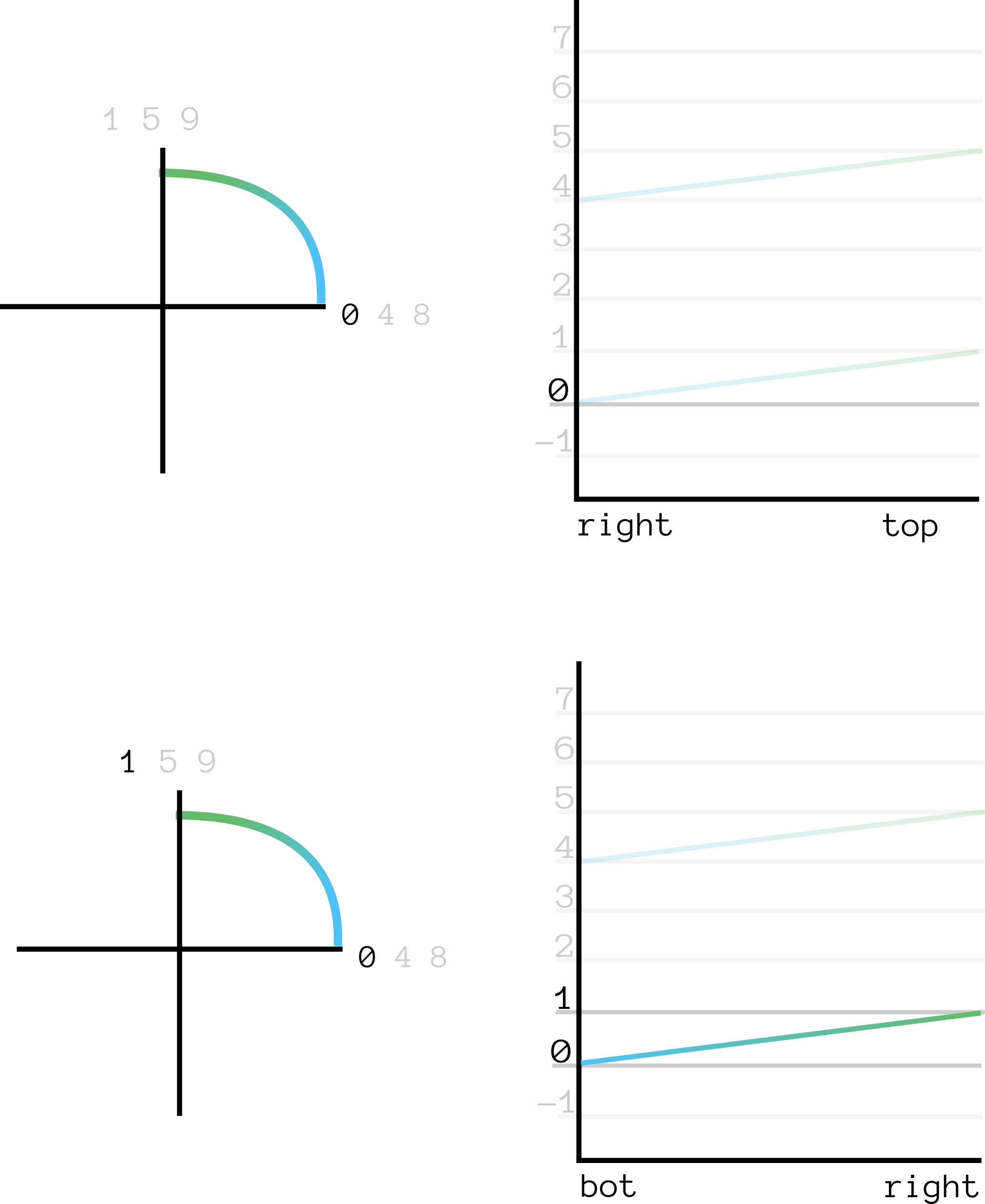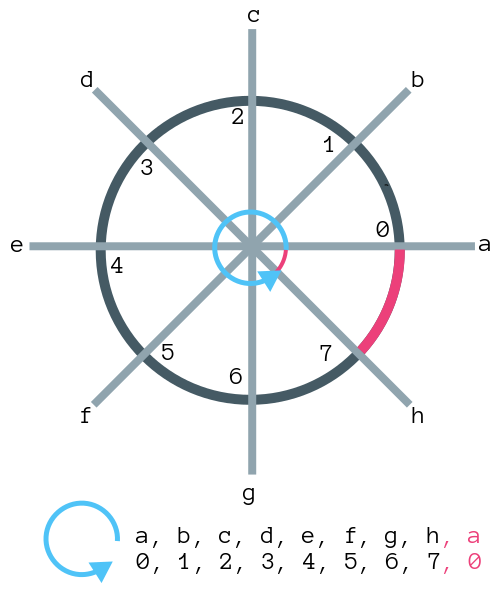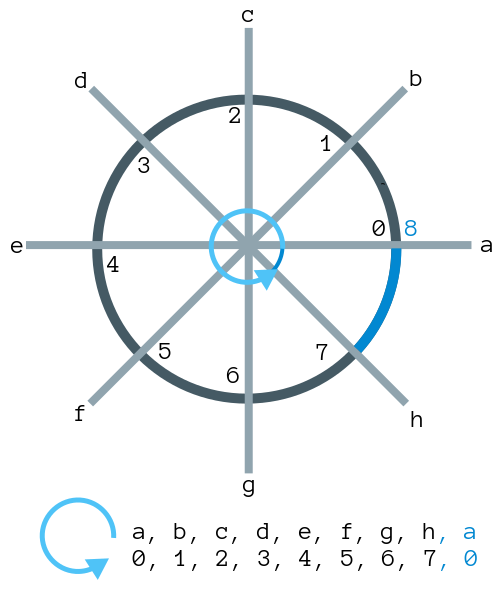## § The arg function, continuity, orientation

Let us think of the function $arg: \mathbb C \rightarrow \mathbb R$ as a multi valued function, which maps each complex number to the set of possible valid angles that generate it:
$arg(z) \equiv \{ t \in \mathbb R : |z|e^{i (\pi/2)t} = z \}$
We plot the function here:• Note that for every value $z \in C$, we get a set of values associated to it.

#### § Recovering single valued-ness

Now, the question is, can we somehow automatically recover single valued-ness? kind of, by stipulating that for any given curve $c: [0, 1] \rightarrow \mathbb C$, the function $arg \circ c: [0, 1] \rightarrow \mathbb R$ is continuous . Let's try to investigate what happens if we move from right towards bot, arbitrarily stipulating ("picking a branch") that arg(right) = 0 as a sort of basepoint.• Note that we were forced to pick the value arg(bot) = -1 from our considerations of continuity. No other value extends continuous from the right to the bottom.
• Also note that we got a smaller value: we move from 0 -> -1: we decrease our value as we move clockwise.
This prompts the natural question:
what happens if we move in the opposite direction?

#### § Counter-clockwise movement

• Let's move counter-clockwise from right, arbitrarily picking the branch arg(right) = 0 as before. This gives us:• Note that once again, we were forced to pick arg(top) = 1 by continuity considerations.
• Also note that this time, we got a larger value: we move from 0 -> 1: we increase our value as we move counter-clockwise

#### § Multiple winding

the true power of this multi-valued approach comes from being able to handle multiple windings. Here the real meaning of being a multi-valued function shows through. If we decide to go through the the loop twice , as:
bot -> right -> top -> left -> bot -> right -> top -> left
-1  -> 0     -> 1   -> 2    -> 3   -> 4     -> 5   -> 6

That is, we end up with the value 6, which can only b

#### § Orientation from continuity

There's something really elegant about being able to recover a notion of "orientation" by simply:
1. Allowing multi-valued functions.
2. Forcing continuity constraints.
3. Interpreting increase/decrease in the value of the function.

#### § Discretizing, gaining more insight

I was personally dis-satisfied with the above explanation, because it seemed weird that we would need to depend on the history to define this function. We can formalize this notion of history. Let's first discretize the situation, giving us:• We are on the space of the spokes, given by a, b, c, d, e, f, g, h.
• We have a function f: Spoke -> Val whose values are given on the spokes.
• We are interested in the path p: Time -> Spoke, p = [a, b, c, d, e, f, g, h, a].
• If we evaluate the function f on the path p, we get out: Time -> Val, out = [0, 1, 2, 3, 4, 5, 6, 7, 0].
• We have a "jump" from 7 to 0 in out as we cross from h to a. This is a discontinuity in out at time 7.
• We want to fix this, so we make the function f multi-valued.• We assign both values 8 and 0 to the spoke a. We wish to define the evaluation of f: Spoke -> 2^N relative to path p. At time t, point p[t], we pick any value in f(p[t]) that makes out[t] continuous.
• So in this case, when we start, we have two choices for out = f(p) = f(a): 0 and 8. But we know that out = f(p) = f(b) = 1. Hence, for out to be continuous, we must pick out = 0.
• Similarly, at out we have two choices: 0 and 8. But we have that out = 7, hence we pick out = 8.
• Note that we say 'we pick the value' that makes out continuous. This is not really rigorous. We can fix this by re-defining f in such a way that f is not Spoke -> Val, but rather it knows the full path: f': (Time -> Spoke) -> Val.

#### § Making the theory path-dependent

path: Time -> Spoke
f: Spoke -> 2^Val -- multi-valued

-- | morally, not mathematically.
out: Time -> Val
out t = choose_best_value (f(path[t]))

But there was a vagueness in this choose_best_value. So we redefine it:
path: Time -> Spoke
f': (Time -> Spoke) -> Time -> Val
f'(path, tcur) =
argmax (\v -> |v - path[tcur-1]| + |v - path[tcur+1|)
f(path[tcur])

out: Time -> Val
out = f'(path)

• The function f' that defines the value of the path has full access to the path itself!
• At time tcur, it attempts to pick the value in f(path[tcur]) which makes the discontinuity as small as possible. It picks a value v from the possible values of f(path[tcur]). This v minimises the of the distances from the previous time point ( |v - path[tcur-1]), and the distance from the next time point ( |v - path[tcur + 1]).
• This provides a rigorous definition of what it means to "pick a value in the branch". This can clearly be extended to the continuous domain.